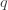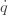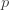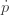Wednesday, November 5, 2014

Multivector momentum

[update 2014-12-10: This post is definitely not quite right. I'm trying to understand units and torsors better. Also, how then to fit heat/temperature better in with the others. Hopefully I'll write about these things one day, but fixing this post seems likely to remain beyond me.]

I trained as a Mathematician, and I try at times to understand some developments in modern Mathematics. Mostly I do this by following John Baez who writes beautifully so that you imagine that you understand it. I’m only vaguely following his current interest in networks, but I loved this diagram (from http://johncarlosbaez.wordpress.com/2013/04/23/network-theory-part-29/) for an unrelated reason:
 displacement:flow:momentum:effort:Mechanics: translation position velocity momentum force Mechanics: rotation angle angular velocity angular momentum torque Electronics charge current flux linkage voltage Hydraulics volume flow pressure momentum pressure Thermal Physics entropy entropy flow temperature momentum temperature Chemistry moles molar flow chemical momentum chemical potential
The first row (after the headings) is the familiar fact that a moving body has momentum which keeps it moving in a straight line at constant speed. To change that requires effort: the application of a force. We are equally familiar in life with the fact that a spinning body (such as a top, or the Earth) has angular momentum which keeps it spinning, and you have to put in effort to stop it.
In the 4th line Dr Baez is thinking of liquids in pipes, but I am more interested in unconstrained gases. If you imagine an explosion then that has pressure momentum which will keep it expanding forever. It requires effort to stop the expansion and that effort would be pressure.
I also want another row which might be the same as Dr Baez’s 6th line. At any rate the momentum in this case is heat. Things will keep the same amount of heat unless some effort (heating/cooling) is applied to change it. This might seem different to the others, since the others are about movement, but actually heat is just internal movements within the matter. The temperature of a gas is proportional to the kinetic energy per molecule.
Now I need to take a detour before I can put these together.

Multivectors

A vector is something with magnitude and direction. Speed is just a number, but velocity (and momentum and force) are vectors because they also include the direction. We imagine vectors as arrows in space with the length representing the magnitude of the vector. You can slide them around: they don’t start at a particular place. Our vectors are three dimensional: every vector can be made of a bit of x, a bit of y and a bit of z.
You can combine two vectors to make a bivector. This is called the exterior product. To imagine this we place the 2nd vector so that it starts where the first finishes. This makes a little parallelogram floating in space, and the area is the magnitude of the bivector. However the bivector is not really a parallelogram: it can be any shape in that plane with that area. And there is a natural way of adding bivectors and they are also 3 dimensional: every bivector can be made of a bit of xy, a bit of yz and a bit of zx.
The exterior product of 3 vectors is a trivector. We can visualize this as a bit of volume with the 3 vectors at edges, but once again it is just the amount of volume that counts not the shape. Trivectors are just one dimensional, since each is just a multiple of the 1x1x1 xyz volume element.
There are nice diagrams of all these at Wikipedia’s Geometric Algebra page: http://en.wikipedia.org/wiki/Geometric_algebra.
It turns out to often be a useful idea to combine our 3 dimensions of vectors, 3 of bivectors, 1 of trivector plus 1 more for scalars (scalars are just numbers). This makes an 8 dimensional space of multivectors. These are mostly particularly useful when combined with a way to multiply them, but we won’t get to that here.

Momentum types

Ordinary momentum is a vector. It has magnitude and direction.
Angular momentum is best seen as a bivector. The area of the bivector is the magnitude, and its orientation (perpendicular to the axis of rotation) determines the rotational geometry.
Since pressure acts in all directions at once, it is natural to see it as a trivector.
Finally we have heat which is intrinsic to the matter and not involved in directions. It is just a scalar value.
It is easy to combine these together into a single multivector value. The question is how meaningful that is.
One aspect is this: Are there other mechanical momentums that this leaves out? I conjecture that for an infinitesimal amount of matter this is all there is.
Speaking of infinitesimal amounts of matter: It is tempting to think that a small amount of matter can’t have much angular momentum. But actually the smaller matter is, the faster it is able to rotate. Even electrons have significant spin (though the mechanicalness of that might be in some doubt). I wonder if the study of fluid mechanics takes adequate account of small rapidly spinning vortices?

Implications

It seems cute, but that is not a justification. It does suggest two lines of investigation:
One is to study fluid mechanics in full generality starting with infinitesimal amounts of matter having these 4 types of momentum. The hope will be that some of the research on Clifford Analysis will turn out to be useful.
The other is to use this in computer simulations of oceans, atmosphere or other fluid situations. These are typically done by dividing the matter to be simulated into little cuboids. The cuboids have some physical characteristics, and the value at the next step is determined from the current value plus the values in neighboring cuboids (plus other forcings, i.e. effort, that may be specified). The hypothesis is that this 8 dimensional value, across 4 types of momentum, is the optimal choice for the value to be stored in each cuboid.